Next: General Overview of the Up: CCD Reductions Previous: Introduction

# Nature of CCD Output

The nominal output Xij of a CCD-element to a quantum of light Iij can be given as(3.1)

where the additive contribution Aij is caused by the dark current, by pre-flashing, by charge that may have skimmed from columns having a deferred charge (skim) and by bias added to the output electronically to avoid problems with digitizing values near zero. Quantum and transfer efficiency of the optical system enter into the multiplicative term M. The term I consist of various components: object, sky and the photons emitted from the telescope structure. It is known that the response of a CCD can show non-linear effects that can be as large as 5-10%. These effects are represented by the term Fij.

In the following we ignore the pre-flash and skim term, and hence only take the bias and dark frames into account. The objective in reducing CCD frames is to determine the relative intensity Iij of a science data frame. In order to do this, at least two more frames are required in addition to the science frame, namely:

• dark frames to describe the term Aij, and
• flat frames to determine the term Mij.

The dark current dark is measured in absence of any external input signal. By considering a number of dark exposures a medium <dark> can be determined: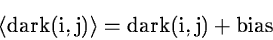(3.2)

The method to correct the frame for multiplicative spatial systematics is know as flat fielding. Flat fields are made by illuminating the CCD with a uniformly emitting source. The flat field then describes the sensitivity over the CCD which is not uniform. A mean flat field frame with a higher S/N ratio can be obtained buy combining a number of flat exposures. The mean flat field and the science frame can be described by: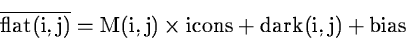(3.3)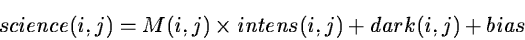(3.4)

where intens(i,j) represents the intensity distribition on the sky, and icons a brightness distribution from a uniform source. If set to the average signal of the dark corrected flat frame or a subimage thereof: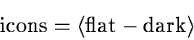(3.5)

then the reduced intensity frame intens will have similar data values as the original science frame science.

Combining Eqs.(3.2), (3.3) and (3.4) we isolate: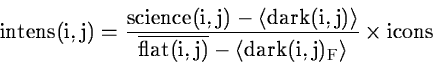(3.6)

Here icons can be any number, and termnow denotes a dark frame obtained by e.g. applying a local median over a stack of single dark frames. The subscript in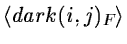denotes that this dark exposures may necessarily be the same frame used to subtract the additive spatial systematics from the raw science frame.

The mean absolute error of intens(i,j) yields with icons = 1 (only the first letter is used for abbreviations):(3.7)

Computing the partial derivatives we get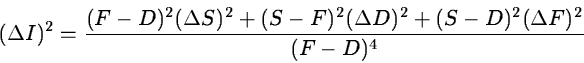(3.8)

A small error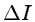is obtained if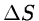,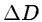and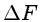are kept small. This is achieved by averaging Dark, Flat and Science frames.is further reduced if S=F, then Equation (3.8) simplifies to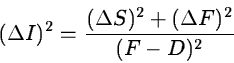(3.9)

This equation holds only at levels near the sky-background and is relevant for detection of low-brightness emission. In practice however it is difficult to get a similar exposure level for the flatfrm and science since the flats are usually measured inside the dome. From this point of view it is desirable to measure the empty sky (adjacent to the object) just before or after the object observations. In the case of infrared observations this is certainly advisable because of variations of the sky on short time scales.Next: General Overview of the Up: CCD Reductions Previous: Introduction
Petra Nass
1999-06-15